# Number Resources

Coding Number Mathematical Modelling Financial Literacy
All 1 2 3 4 5 6 7 8
Overall Lesson Webinar Recording Lesson Support
Select All    Clear All

NAME
CATEGORY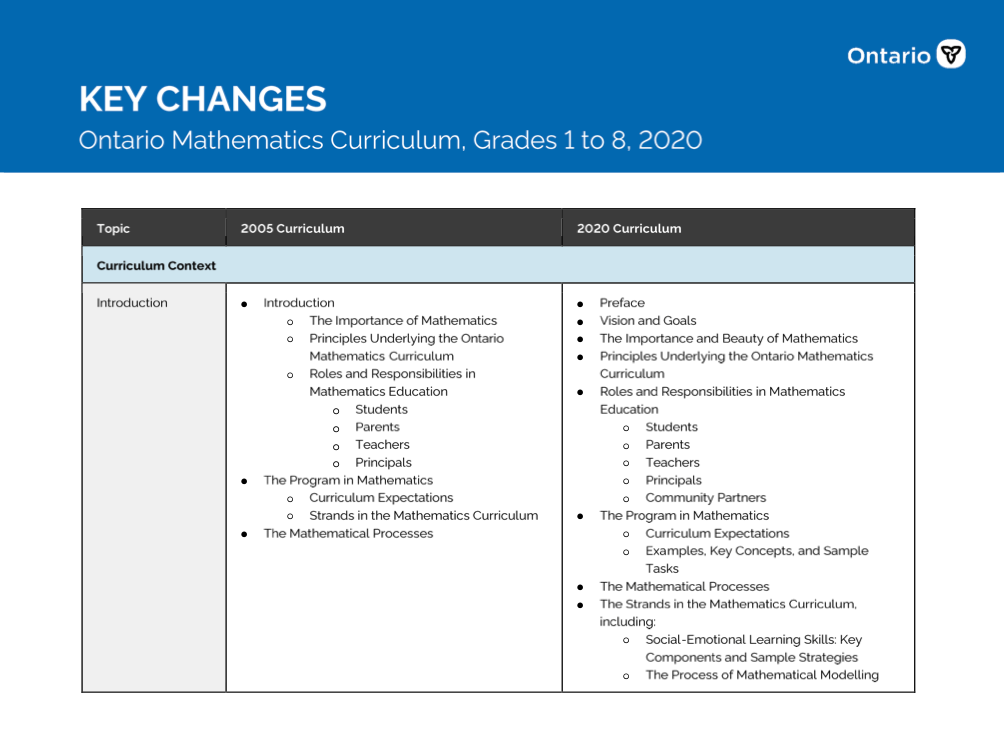Key Changes - Ontario Mathematics Curriculum, Grades 1 to 8, 2020   [PDF]

This document, prepared by the Ontario Ministry of Education, compares in table form the key changes between the 2005 and the 2020 elementary mathematics curriculum.

Overall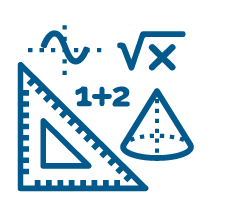Ontario Mathematics Curriculum, 2020, Grades 1 - 8   [URL]

This curriculum policy replaces The Ontario Curriculum, Grades 1-8: Mathematics, 2005. Beginning in September 2020, all mathematics programs for Grades 1 to 8 will be based on the expectations outlined in this curriculum policy.

Overall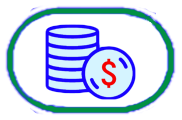Gr 7/8 Webinar: Financial Literacy in the Intermediate Grades   [MOV]

Welcome to this webinar about teaching the new Financial Literacy expectations for the intermediate grades of the revised mathematics curriculum. In this webinar, we take you through the lessons that have been created by Ontario educators to support Ontario educators. In developing the Financial Literacy lessons for this project, the intentional decision to use scenarios was made. Scenarios give the opportunity to have a discussion about financial choices and the inequities of access to those choices. The goal was to provide students with a chance to learn and understand the individual skills needed that impact personal financial choices and also the economic literacy needed to understand the impact of financial systems as a whole.

Webinar Recording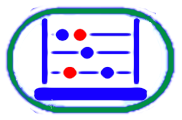Gr 7/8 Webinar: Fractions in the Intermediate Grades   [MOV]

Welcome to this webinar about teaching the Number expectations for the intermediate grades of the revised mathematics curriculum. In this webinar, we take you through the lessons that have been created by Ontario educators to support Ontario educators. These Number lessons have a focus on developing fraction concepts and skills. These lessons benefit from the latest research about learning fractions, including an emphasis on high-yield visual representations.

Webinar RecordingGr 4/5/6 Webinar: Fractions in the Junior Grades   [MOV]

Welcome to this webinar about teaching the Number expectations for the junior grades of the revised mathematics curriculum. In this webinar, we take you through the lessons that have been created by Ontario educators to support Ontario educators. These Number lessons have a focus on developing fraction concepts and skills. These lessons benefit from the latest research about learning fractions, including an emphasis on high-yield visual representations.

Webinar RecordingGr 1/2/3 Webinar: Fractions in the Primary Grades   [MOV]

Welcome to this webinar about teaching the Number expectations for the primary grades of the revised mathematics curriculum. In this webinar, we take you through the lessons that have been created by Ontario educators to support Ontario educators. These Number lessons have a focus on developing fraction concepts and skills. These lessons benefit from the latest research about learning fractions, including an emphasis on high-yield visual representations.

Webinar RecordingGrade 1 - Mathematical Modelling Lesson - Goody bags for a birthday party   [Folder]

In this lesson, children learn to make a mathematical model in order to predict the number of candies required for a candy bag. Major foci include determining important questions that need answering, missing information that is needed to solve the problem, and making assumptions about missing information.

LessonGrade 1 - Number Lesson - Dancing on Fractions   [Folder]

In this lesson, students learn to divide different shapes and objects into equal parts to share, use math language like halves and fourths to describe equal parts, and understand that more equal shares creates smaller shares.

LessonGrade 1 - Number Lesson - Class Visit to the Zoo   [Folder]

The class is going on an imaginary visit to the zoo. During the visit, the class is going to feed some of the animals. The class has a limited budget so they can only buy a limited amount of snacks. They can only feed 2 giraffes, 4 lions, 2 chimpanzees, 4 zebras and 2 elephants. Students work in pairs or small groups to decide how to share food fairly among each type of animal making sure to respect the snack preferences of the animals.

LessonGrade 1 - Number Lesson - Feeding Bears at the Zoo   [Folder]

The students use their imagination to pretend that they are going to the zoo for a day to work with the zookeepers as assistants. One of their jobs is to divide a specific amount of food equally among the 2 polar bears. They also have to divide the same amounts among 4 grizzly bears and among 10 black bears.

LessonGrade 1 - Number Lesson - Building Animal Spaces at the Zoo   [Folder]

In this lesson, students imagine that a zoo is bringing in some new animals and that they are in charge of designing and building new enclosures for them. The challenge is that there is limited space. Students use their knowledge of fractions ot design the new enclosures.

LessonGrade 2 - Coding Lesson - Building Number Sentences   [Folder]

In this lesson, students use coding devices to do plugged sequential coding to create number sentences while using a 100's chart.

LessonGrade 2 - Coding Lesson - Coding for the squirrel   [Folder]

In this lesson, students will read a story and help the squirrel to find, share and store acorns in the forest, and to problem solve using coding skills.

LessonGrade 2 - Financial Literacy Lesson - \$100 for Recess Equipment   [Folder]

In this lesson, students will calculate amounts of up to \$100 for recess equipment.

LessonGrade 2 - Financial Literacy Lesson - \$200 for Recycling   [Folder]

In this lesson, students will find several ways to represent \$ 200 in order to decide what currency they would like to receive from the city for their recycling materials.

LessonGrade 2 - Mathematical Modelling Lesson - Organizing a Math Manipulatives Area   [Folder]

In this lesson, students will work on the 2 first components of the math modeling process. They will determine how to organize the math manipulatives area for a grade 2 classroom.

LessonGrade 2 - Mathematical Modelling Lesson - Planning an event   [Doc]

In this lesson, students learn to understand the first 2 components of mathematical modeling. They develop a spatial plan for the grade 2 event in the schoolyard.

LessonGrade 2 - Number Lesson - Split, the squirrel, shares his seeds   [Folder]

Split, the squirrel, shares his seeds - in this lesson, students learn to create equivalent sharing situations of one-third and two-sixths between 3 squirrels.

LessonGrade 2 - Number Lesson - Wooly and her sweaters!   [Folder]

In this lesson, Wooly has her Grandma knit sweaters with different combinations of coloured stripes on sweaters to represent one half, two-fourths, one third and two-sixths (surface model). She then knits her scarves with pompoms having the same fraction quantities as the sweaters (set model). The equivalent fractions are then compared for each model. Students represent all of these fractions with different manipulatives. The photos from the centers can then be used for different card games.

LessonGrade 2 - Number Lesson - Let's Create Art with Modeling Clay   [Folder]

In this lesson, students represent multiplication as repeated addition of equal groups, including groups of one-half and one-fourth, and determine the amound of clay needed to buy for the class to create art. They use various tools and drawings to solve the problem.

LessonGrade 2 - Number Lesson - Dried Fish to Share   [Folder]

In this lesson, students represent and solve problems of equitably sharing a whole of up to 10 dried fish among a number of people. The results are mixed numbers. Links to aboriginal activities are presented as a context.

LessonGrade 3 - Coding Lesson - Making Change   [Folder]

In this lesson students will have opportunities to estimate change using coins as well as debug code. It explores financial literacy as well as coding.

LessonGrade 3 - Financial Literacy Lesson - The Missing Penny   [Folder]

In this lesson students work on rounding to the nearest 5 and 10 and make change for amounts under a dollar. It explores how pennies are used in cash and virtual transactions and provides an opportunity to discuss the role of the penny.The Missing Penny is the first of two lessons. The companion lesson, Making Change, is the second lesson in this duo. Making Change is found in the Coding lessons.

LessonGrade 3 - Financial Literacy Lesson - The Value of Small Change   [Folder]

This lesson looks at what the word value means and how that relates to money. Students explore whether small change has value. In this lesson, students estimate and find the value of collection of coins and estimate and calculate the change from cash transactions for amounts less than one dollar.

LessonGrade 3 - Number Lesson - On your mark, get set, go!   [Folder]

In this lesson, students explore the world of fractions and equivalent fractions through a bicycle race using a three-act task. It provides an opportunity to represent fractions using a linear model. Some games are also included to help students consolidate the concept of fractions using different representations.

LessonGrade 3 - Number Lesson - Doggie Treats   [Folder]

This lesson provides opportunities to work with equivalent fractions while solving fair share problems. Students are encouraged to explore a range of fractional units while reinforcing the importance of equal parts and using multiple ways to name or represent fractions.

LessonGrade 3 - Number Lesson - Thank You cards   [Folder]

In this lesson, students will have the opportunity to create a thank you card while applying fraction concepts. They will first have to divide their card using halves, thirds and fourths. Then they will determine the number of stickers to use to decorate their card as a fraction of the set of stickers..

LessonGrade 4 - Financial Literacy Lesson - Becoming an Informed Consumer / Does this Deal Make Sense to You ?   [Folder]

Use estimation strategies and operations to determine the best price for bundled items by finding unit rate.

LessonGrade 4 - Mathematical Modelling Lesson - Planning the School Breakfast Club   [Doc]

This lesson is the first of two. Students begin the first three steps of the mathematical modeling process: understanding the situation, analyzing the situation, and creating a mathematical model. From an authentic activity, students work together to come up with a simple model and collect the data. This lesson will be followed by a second lesson called Let's Serve Breakfast! where students continue the mathematical modeling process.

LessonGrade 4 - Mathematical Modelling Lesson - Breakfast is served!   [Doc]

In this lesson, students continue to analyze, evaluate, and modify as necessary their mathematical model presented in Lesson 1 (Planning the School Breakfast Club). They have the opportunity to collect and interpret additional data to answer further questions, create new models, and seek practical solutions to the given situation.

LessonGrade 4 - Number Lesson - Draw It: Fraction Discoveries   [Folder]

Create drawings to understand the meaning of the numerator and denominator in fractions written in standard notation.

LessonGrade 4 - Number Lesson - How Many Books?   [Doc]

In this lesson, students will have the chance to compare fractions in two different fair-share scenarios. They can compare them by using drawings and models to compare them.

LessonGrade 4 - Number Lesson - Combining Fractions   [Doc]

In this lesson, students will count unit fractions to help them represent the relationship between repeated addition of unit fractions and multiplication of those same unit fractions by a whole number.

LessonGrade 5 - Coding Lesson - Recalling Math Facts through Coding   [Folder]

In this lesson, students will learn how to recall and demonstrate multiplication and division facts up to 12 x 12 by using and manipulating existing code. They will apply this code to a spreadsheet to create original artwork.

LessonGrade 5 - Coding Lesson - Exploring Patterns with fractions Using Scratch   [Doc]

In this lesson, using Scratch, students will learn to understand and represent patterns, relating them to equivalent decimals and fractions so they understand how the size of the whole affects the size of a fraction. They will also learn to make predictions about patterns.

LessonGrade 5 - Financial Literacy Lesson - Budgeting and Goal Setting   [Folder]

In this lesson, students will begin to think about budgets. Teachers can choose between 2 scenarios (raising money for a trip, or earning money from a job like babysitting) to explore. Students will consider why they may want to earn money, how they might earn it, and prioritize how it might be spent.

LessonGrade 5 - Financial Literacy Lesson - Estimating Costs including HST   [Folder]

In this lesson, students will learn how to estimate and calculate total costs (including HST) and compare that to how much money they have. They will "visit" restaurants to explore estimating and then actually calculate costs given menu items.

LessonGrade 5 - Financial Literacy Lesson - Unit Rates   [Folder]

In this lesson, students learn to compare prices for different amounts of a product. Many items are sold in packs of various sizes. What is the best price? Are there other factors that influence what you decide to buy? Students learn about various strategies, including finding unit rates, to make financial decisions.

LessonGrade 5 - Mathematical Modelling Lesson - Is All Screen Time Created Equally?   [Folder]

In this lesson, students consider the idea of screentime. Many experts think children should limit screentime, and parents want to support their children to start making good choices about amounts of screentime, and what activities might be more or less valuable. Students engage in the process of mathematical modelling to develop a tool that parents and children could use to monitor their screentime, and make good choices about how to spend that time.

LessonGrade 5 - Mathematical Modelling Lesson - Greenhouses in the Arctic   [Folder]

In this lesson, students will consider the question of how fresh produce be reliably supplied to people living in remote Arctic communities. They will consider issues such as transportation costs, the feasibility of building greenhouses, and living in remote communities.

LessonGrade 5 - Number Lesson - Interactive Fractions, Decimals and Percentages   [Folder]

In this lesson, students explore the interrelationship between fractions, decimals and percentages. Students navigate an online interactive Google Slide that allows to learn and explore all while using visuals and digital manipulatives.

LessonGrade 5 - Number Lesson - Multiply a Unit fraction by a Whole number   [Folder]

In this lesson, students will learn how to multiply fractions by a whole number using a variety of strategies. They will also decide which strategies they prefer. Students will use concrete materials and other visual tools to help them understand the different strategies for solving questions.

LessonGrade 5 - Number Lesson - Represent and Compare Fractions   [Folder]

In this lesson, students explore various fraction representations (area model, set model and number line model). Then, they are challenged to use those models to represent and solve several problems that require them to compare fractions, including mixed and improper fractions.

LessonGrade 6 - Coding Lesson - Part 1: Getting Ready to Code Transformations - Unplugged!   [Folder]

In an unplugged environment, students will be learning to understand the movement of objects on the Cartesian plane through the graphing of ordered pairs (x, y) and using combinations of translations and reflections to transform objects. This lesson can be used in advance of Lesson 1, "Coding Transformations - Unplugged!"

LessonGrade 6 - Coding Lesson - Part 2: Coding Transformations - Unplugged   [Folder]

In an unplugged environment, students will be coding transformations--combinations of translations and reflections--on the Cartesian plane by writing and executing pseudocode.

LessonGrade 6 - Coding Lesson - Part 1: Which Integer is Greater?   [Folder]

Creating a pseudocode for the actions to determine which integer is greater than another, students then identify the Scratch coding blocks that will carry out these actions. They can remix a sample Scratch project or programme their own from a starter Scratch project. The use of a number line in the coding projects gives a visual representation to the integers. Note: For teachers not familiar with Scratch coding, instructions are included to assist your teaching of these lessons.

LessonGrade 6 - Coding Lesson - Part 2: Mystery Integers!   [Folder]

Creating a pseudocode for the actions to determine the actions used when using an "input/output machine" students then identify the Scratch coding blocks that will carry out these actions. They can remix a sample Scratch project or programme their own from a starter Scratch project. This lesson and Which is Greater? focus on developing your students' understanding of positive and negative integers. Note: For teachers not familiar with Scratch coding, instructions are included to assist your teaching of these lessons.

LessonGrade 6 - Coding Lesson - Start Small - Making Informed Financial Decisions   [Folder]

In this lesson, students will code using Google Sheets or any spreadsheet program. Students will be creating a budget to help plan for a financial goal while considering earnings and expenses.

LessonGrade 6 - Coding Lesson - Coding and Composite Numbers   [Folder]

Through the use of pseudocode, students will be learning to decompose their mathematical thinking regarding the concepts of prime and composite numbers and algorithms; identify the factors of composite numbers as a series of actions; connect those actions to block coding, and in doing so, reinforce their computational thinking. Note: Consider using coding lesson after the students understand the concept of factoring. For teachers not familiar with Scratch coding, instructions are included to assist your teaching of these lessons.

LessonGrade 6 - Financial Literacy Lesson - In the Interest of Saving!   [Folder]

In this lesson, students will be learning to compare different methods of payment--identifying advantages and disadvantages--and the best ways to use them. Students will also analyze and identify how interest rates are applied to various methods of payment in order to make the best financial decision possible when making a purchase.

LessonGrade 6 - Number Lesson - It All Adds Up! Linear Models for Fraction Sums   [Folder]

Students will use visual and concrete models, including number lines, to further develop their reasoning about how fractions can be combined through addition.

LessonGrade 6 - Number Lesson - All Tied Up About Fractions   [Folder]

In this lesson, students will use concrete manipulatives, online apps, and simulations to examine fraction relationships. The Active Learning component centres on situations about the manufacturing process of shoelace making. Further lesson consolidation includes visual art ideas for patterns and tessellations.

LessonGrade 6 - Number Lesson - It's Going to be a Banner Day!   [Folder]

In this lesson, students will be learning to multiply and divide proper fractions by whole numbers by calculating the amount of fabric used during the creation of class banners.

LessonGrade 7 - Coding Lesson - Restaurant Order app   [Folder]

A lesson in which students get to create a prototype for a restaurant ordering app. Using different conditional and operations blocs in Scratch, students get to work on expectations in the Numbers strand and calculate the total cost of a meal. Various scenarios (with different items and different quantities) allow for differentiation and various levels of scaffolding.

LessonGrade 7 - Coding Lesson - \$ 20 or 20%?   [Folder]

In this coding lesson using Scratch, students can create a calculator in which they can compare two different discount structures. Depending on the number of items bought, the calculator will be able to provide savings from either choosing a 20% discount, or a \$ 20 discount. Students get to utilise conditions and operations blocs in this activity.

LessonGrade 7 - Financial Literacy Lesson - Saving for a Smartphone   [Folder]

In this lesson, students will learn about the value of creating a budget, different types of savings accounts and the implications interest rates can have on saving money. Following that, students will use money earned from a part-time job and saved over time to investigate various mobile phone plans to determine which one best suits their needs.

LessonGrade 7 - Mathematical Modelling Lesson - Handwashing Policy   [Folder]

In this lessons students will create a model then collect and organize data in order to determine the impact of a school board's handwashing policy. Students will use their model to make predictions, then test those predictions by playing out the handwashing scenario in real-life. In the end, students will present their opinion on the policy, using mathematical arguements based on information provided by their models.

LessonGrade 7 - Number Lesson - Palace Pizza Catering Company   [Folder]

In this lesson, students work with a fictional pizza recipe to multiply and divide fractions by whole numbers and fractions. Students will then use their understanding of multiplying and dividing fractions by fractions in order to create and solve their own problems.

LessonGrade 7 - Number Lesson - Choosing Operations   [Folder]

In this lesson, students work together to visually represent and solve a variety of problems involving fractions. Students will develop a deeper understanding of when and why they should use addition, subtraction, multiplication and division when working with fractions.

LessonGrade 7 - Number Lesson - Zoo Visit   [Folder]

In this lesson, students use fractions based on minutes in an hour to create a schedule for visiting the zoo. Several scenarios will allow them to work on operations with fractions, equivalent fractions and mixed numbers.

LessonGrade 8 - Coding Lesson - Coding with the Pythagorean Theorem   [Doc]

In this lesson, students will increase their knowledge of the Pythagorean Theorem while working on their coding skills.

LessonGrade 8 - Coding Lesson - Coding YouTube   [Doc]

Do your students want to become YouTube stars? Students will learn how to Monetize a YouTube Channel and use coding to predict the revenue a YouTube video can generate.

LessonGrade 8 - Financial Literacy Lesson - Understanding Interest   [Folder]

In this lesson, student will investigate different aspects of how interest is calculated, charged and earned. Students will compare scenarios to understand the impact of interest.

LessonGrade 8 - Number Lesson - Publicity and the Art of Fraction Manipulation   [Folder]

Are fractions properly used in everyday publicity? Using publicity posters, students will explore fractions and spatial sense.

LessonGrade 8 - Number Lesson - Cake Shop   [Folder]

In this lesson, students learn about dividing fractions by a whole number, a whole number by a fraction, and a fraction by a fraction using drawing and manipulatives.

LessonGrade 8 - Number Lesson - Fraction Target Game   [Folder]

In this lesson, students will practice adding, subtracting, multiplying and dividing fractions by playing games.

Lesson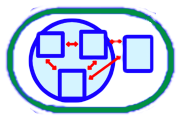Gr 7/8 Webinar: Mathematical Modelling in the Intermediate Grades   [MOV]

Welcome to this webinar about teaching the new Mathematical Modelling expectations for the intermediate grades of the revised mathematics curriculum. In this webinar, we take you through the lessons that have been created by Ontario educators to support Ontario educators. These Mathematical Modelling lessons have a focus on developing students’ appreciation for the role mathematics plays in real “real life” while providing students with opportunities to experience mathematics in a more personal and relevant way.

Webinar RecordingGr 4/5/6 Webinar: Mathematical Modelling in the Junior Grades   [MOV]

Welcome to this webinar about teaching the new Mathematical Modelling expectations for the junior grades of the revised mathematics curriculum. In this webinar, we take you through the lessons that have been created by Ontario educators to support Ontario educators. These Mathematical Modelling lessons have a focus on developing students’ appreciation for the role mathematics plays in real “real life” while providing students with opportunities to experience mathematics in a more personal and relevant way.

Webinar RecordingVirtual Manipulatives for Fractions   [Doc]

A summary of Virtual Manipulatives for Fractions that are available, including links, and considerations for their usage.

Lesson Support